出租广告位,需要合作请联系站长

+关注

## python matplotlib自定义colorbar颜色条-以及matplotlib中的内置色条

### 文章目录

PS: 传送门——自定义Colorbars教程

## 自定义colorbar（draw colorbar without any mapple/plot）

参考：Customized Colorbars Tutorial
api example code: colorbar_only.py
自定义colorbar可以画出任何自己想要的colorbar，自由自在、不受约束，不依赖于任何已有的图(plot/mappable)。这里使用的是mpl.colorbar.ColorbarBase类，而colorbar类必须依赖于已有的图。
关于该类的参数如下 (该类参数描述没找到，部分参数的取值可参考colorbar中的描述)：

class matplotlib.colorbar.ColorbarBase(ax, cmap=None, norm=None, alpha=None, values=None, boundaries=None, orientation=‘vertical’, ticklocation=‘auto’, extend=‘neither’, spacing=‘uniform’, ticks=None, format=None, drawedges=False, filled=True, extendfrac=None, extendrect=False, label=’’)[source]
参数简单描述

ax :可用于设置colorbar的位置、长、宽
norm :用于规范化–设置颜色条最大最小值
cmap：颜色（可参考本篇博文的最后部分——推荐色带与自定义色带）
boundaries：要想使用extend，在norm之外，必须要有两个额外的boundaries
orientation：colorbar方向，躺平or垂直
extend：延伸方向(在norm之外colorbar可延伸)
ticks：自定义各段的tick（记号）

下面是一个例子，可以自己试试调整各项参数：

``````import numpy as np
import pylab as plt
import matplotlib as mpl

if __name__ == '__main__':
fig = plt.figure(figsize=(3, 8))
cmap = mpl.cm.Spectral_r
ax3 = fig.add_axes([0.3, 0.2, 0.2, 0.5]) # 四个参数分别是左、下、宽、长
norm = mpl.colors.Normalize(vmin=1.3, vmax=2.5)
bounds = [ round(elem, 2) for elem in np.linspace(1.3, 2.5, 14)] #
cb3 = mpl.colorbar.ColorbarBase(ax3, cmap=cmap,
norm=norm,
# to use 'extend', you must
# specify two extra boundaries:
boundaries= [1.2] + bounds + [2.6],
extend='both',
ticks=bounds,  # optional
spacing='proportional',
orientation='vertical')
plt.show()
```1234567891011121314151617181920```

## contourf函数

contour和contourf分别绘制轮廓线和填充轮廓线。两个版本的函数签名和返回值是相同的。关于两者画图的一些实例可以参阅python matplotlib contour画等高线图，以及matplotlib中文文档

``````    contourf([X, Y,] Z, [levels], **kwargs) # [ ]表示该参数是可选的——可以给定也可以不给定
```1```

contourf中的基本参数：

X, Y : array-like, optional

contourf参数中X和Y都必须是二维的，形状与Z相同(例如，通过numpy.meshgrid创建)；或者都必须是一维的，这样len(X) == M是Z中的列数，len(Y) == N是Z中的行数。
如果没有给定，则假设它们是整数索引，即X = range(M)， Y = range(N)。

Z : array-like(N, M)

绘制轮廓线的高度值。

levels : int or array-like, optional

确定等高线/区域的数量和位置。
如果是int n，则使用n个数据间隔;即画n+1条等高线。水平高度是自动选择的。
如果类似数组，则在指定的级别上绘制等高线。这些值必须是递增的。

## 为所有子图添加一个色带

参考：Matplotlib 2 Subplots, 1 Colorbar
在有图的情况下，为所有子图添加一个colorbar：

``````import numpy as np
import matplotlib.pyplot as plt

fig, axes = plt.subplots(nrows=2, ncols=2)
for ax in axes.flat:
im = ax.imshow(np.random.random((10,10)), vmin=0, vmax=1)

cbar_ax = fig.add_axes([0.85, 0.15, 0.05, 0.7])
fig.colorbar(im, cax=cbar_ax)

plt.show()
```123456789101112```

## 推荐色带与自定义色带

在画图的时候，可以用推荐色带。在Contourf 与记录颜色刻度中，发现contourf中有cmap参数，cmap即是色带。cmap的值可以参考Colormap reference
下面这段代码来自Contourf 与记录颜色刻度

``````cs = ax.contourf(X, Y, z, locator=ticker.LogLocator(), cmap=cm.PuBu_r) # PuBu_r是PuBu色带的倒转
cbar = fig.colorbar(cs)
```12```

其中cmap就是使用matplotlib提供的色带，如果要自己制定色带可以用参数colors如下：

``````# Colors是一些自选颜色列表
Colors=('#DDDDFF','#7D7DFF','#0000C6','#000079','#CEFFCE','#28FF28','#007500','#FFFF93','#8C8C00','#FFB5B5','#FF0000','#CE0000','#750000')
cs=m.contourf(xi, yi, z, colors=Colors, levels=levels, extend='both')  # 这里m是一个basemap实例
```123```

在cmap中提供了一些默认色带：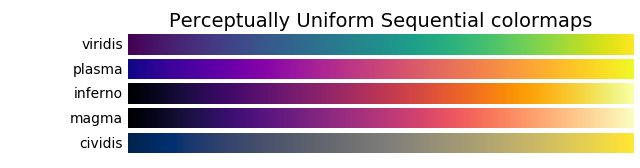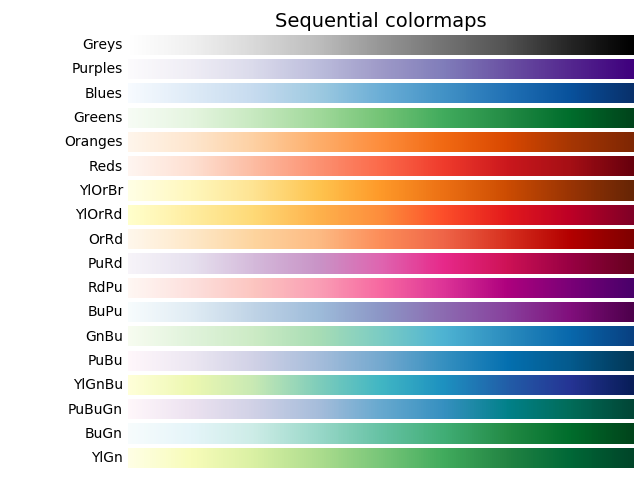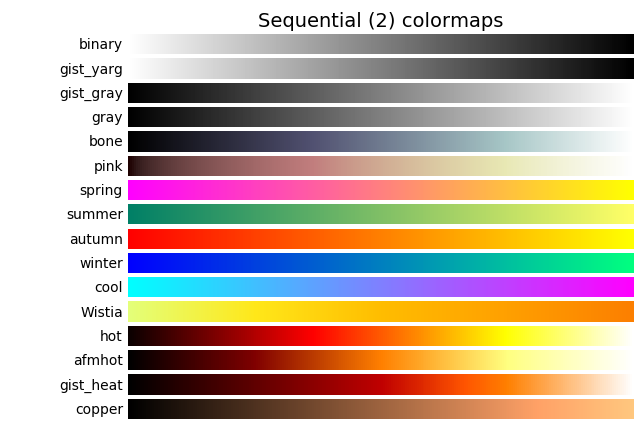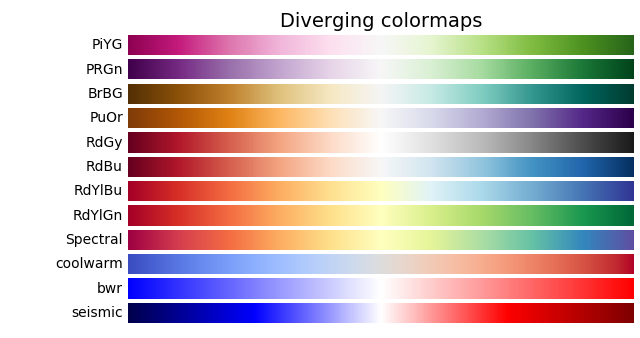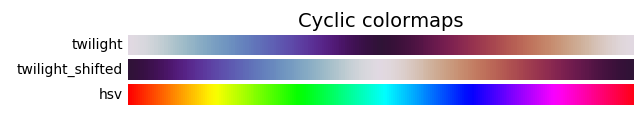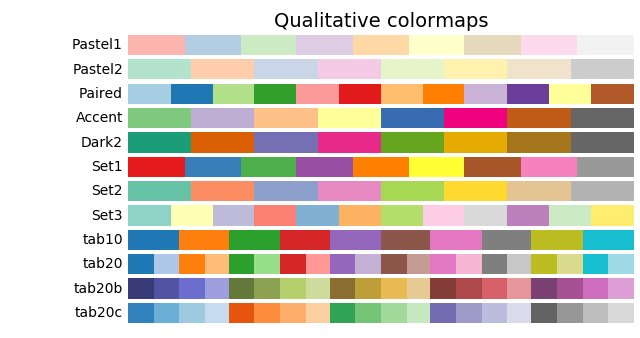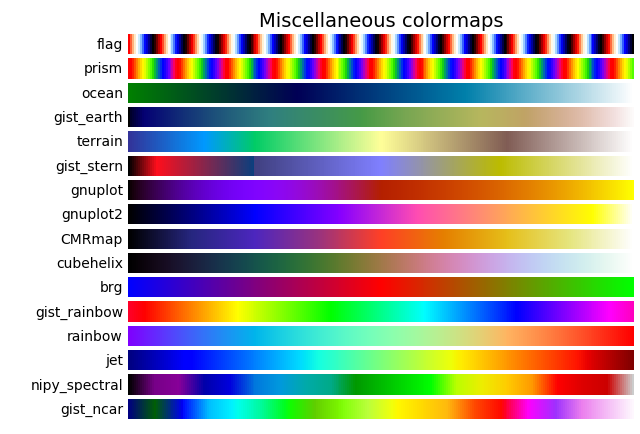关注本站官方公众号:程序员总部，领取三大福利！ 福利一:python和前端辅导 福利二:进程序员交流微信群，专属于程序员的圈子 福利三:领取全套零基础视频教程(python,java,前端,php)
关注公众号回复python,免费领取 全套python视频,回复充值+你的账号,免费为您充值1000积分

所属网站分类: 技术文章 > 博客

作者：dkjf787

来源：python黑洞网

任何形式的转载都请注明出处,如有侵权 一经发现 必将追究其法律责任

1 0
收藏该文
已收藏

评论内容：(最多支持255个字符)

站长公众号(new) 更多>
pdf(new) 更多>
脚本(new) 更多>
博客(new) 更多>
视频(new) 更多>
实战(new) 更多>
问答(new) 更多>
游戏(new) 更多>
其他资源(new) 更多>